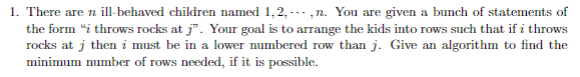# Question Solved1 Answer1. There are n ill-behaved children named 1,2,. ,n. You are given a bunch of statements of the form " throws rocks at j”. Your goal is to arrange the kids into rows such that if i throws rocks at j then i must be in a lower numbered row than j. Give an algorithm to find the minimum number of rows needed, if it is possible.966IXX The Asker · Computer ScienceTranscribed Image Text: 1. There are n ill-behaved children named 1,2,. ,n. You are given a bunch of statements of the form " throws rocks at j”. Your goal is to arrange the kids into rows such that if i throws rocks at j then i must be in a lower numbered row than j. Give an algorithm to find the minimum number of rows needed, if it is possible.
More
Transcribed Image Text: 1. There are n ill-behaved children named 1,2,. ,n. You are given a bunch of statements of the form " throws rocks at j”. Your goal is to arrange the kids into rows such that if i throws rocks at j then i must be in a lower numbered row than j. Give an algorithm to find the minimum number of rows needed, if it is possible.